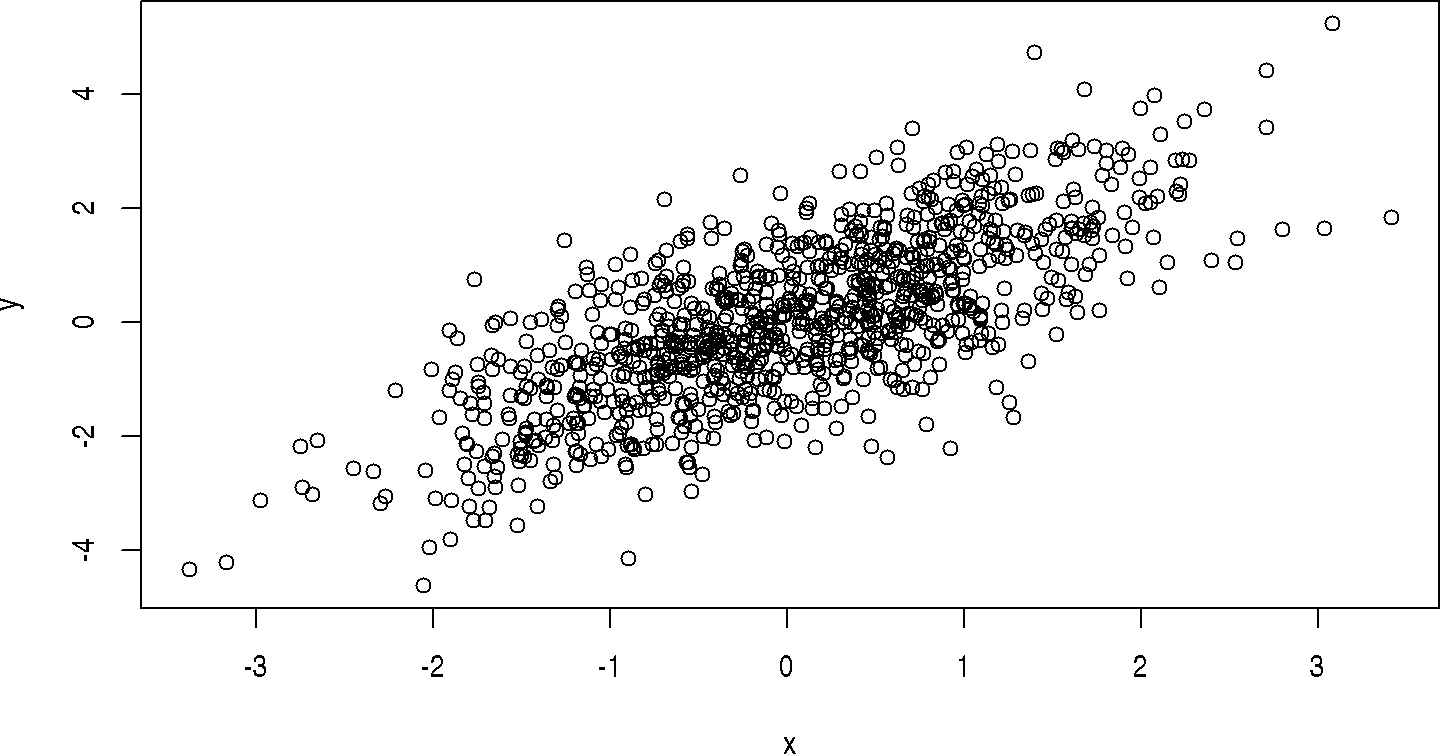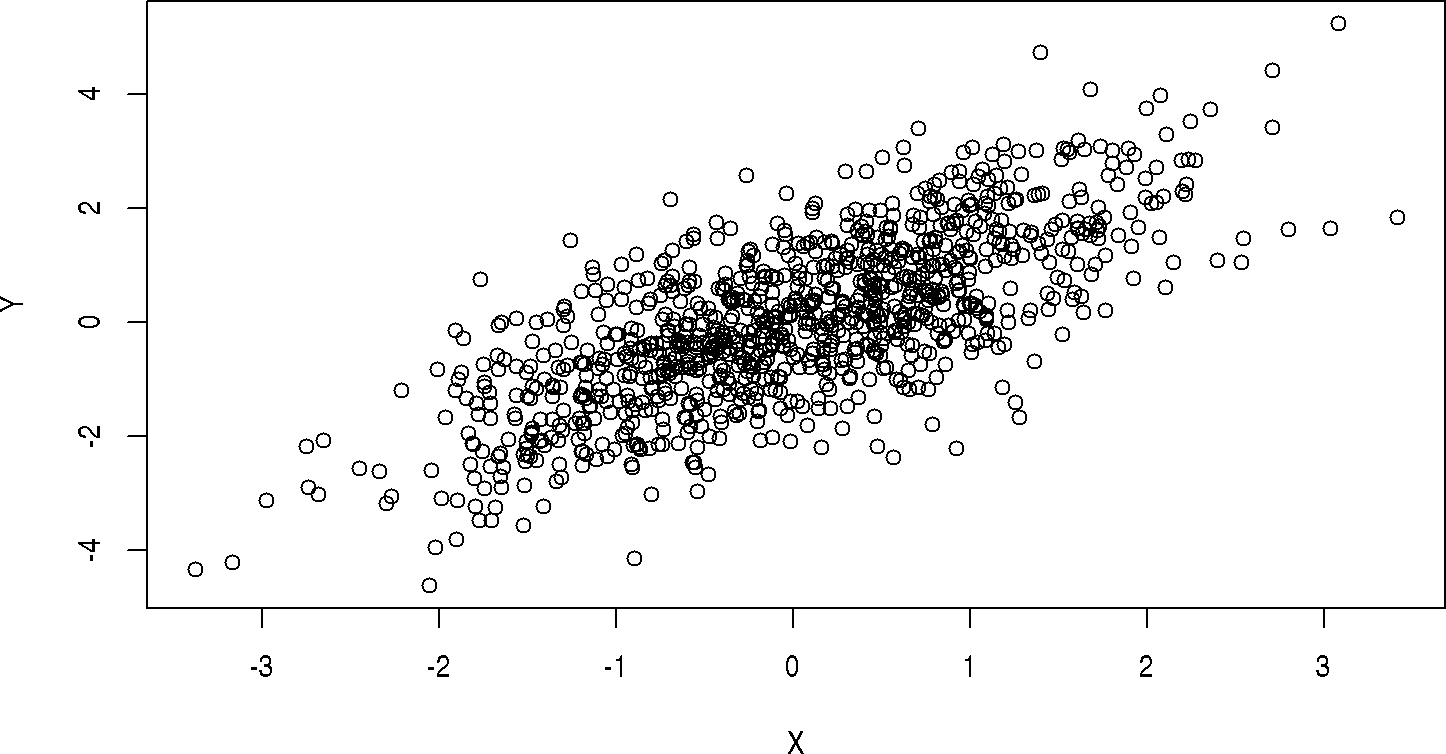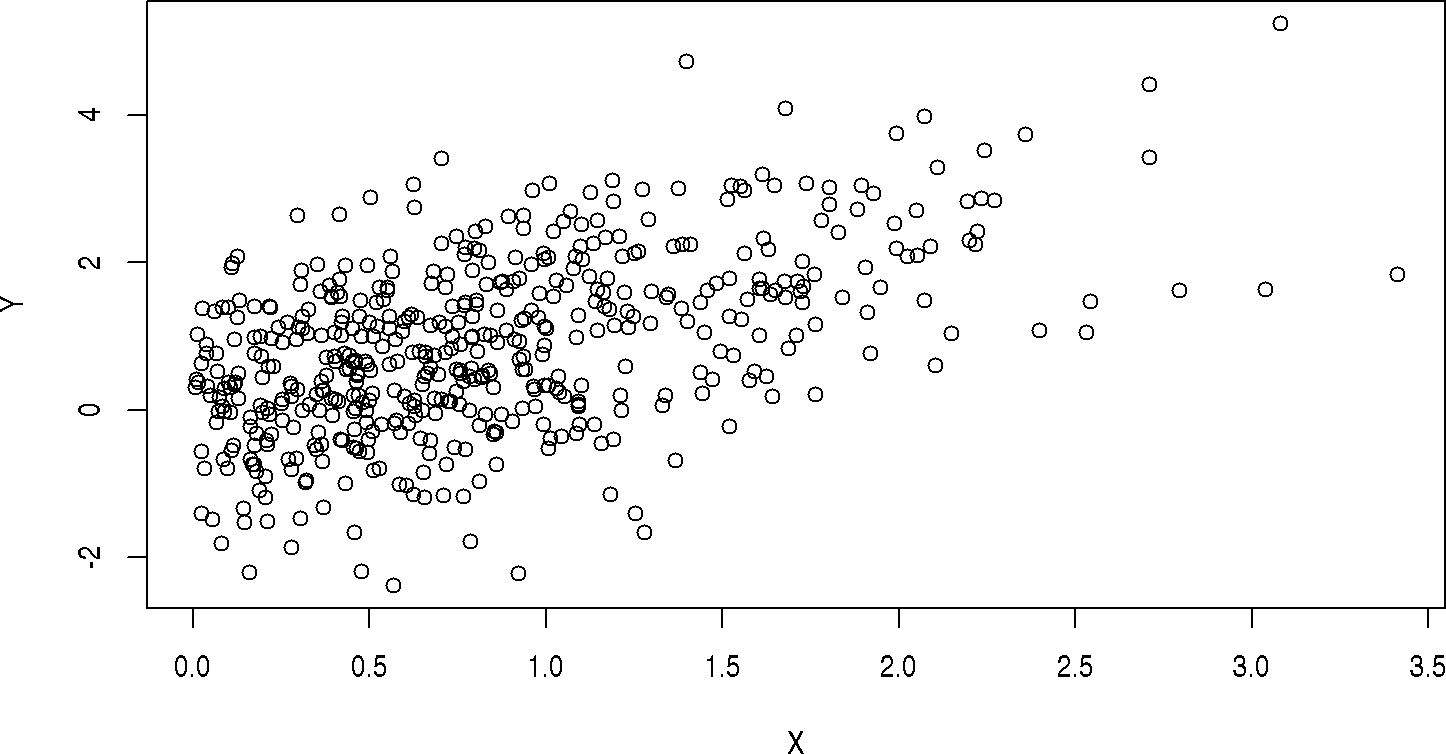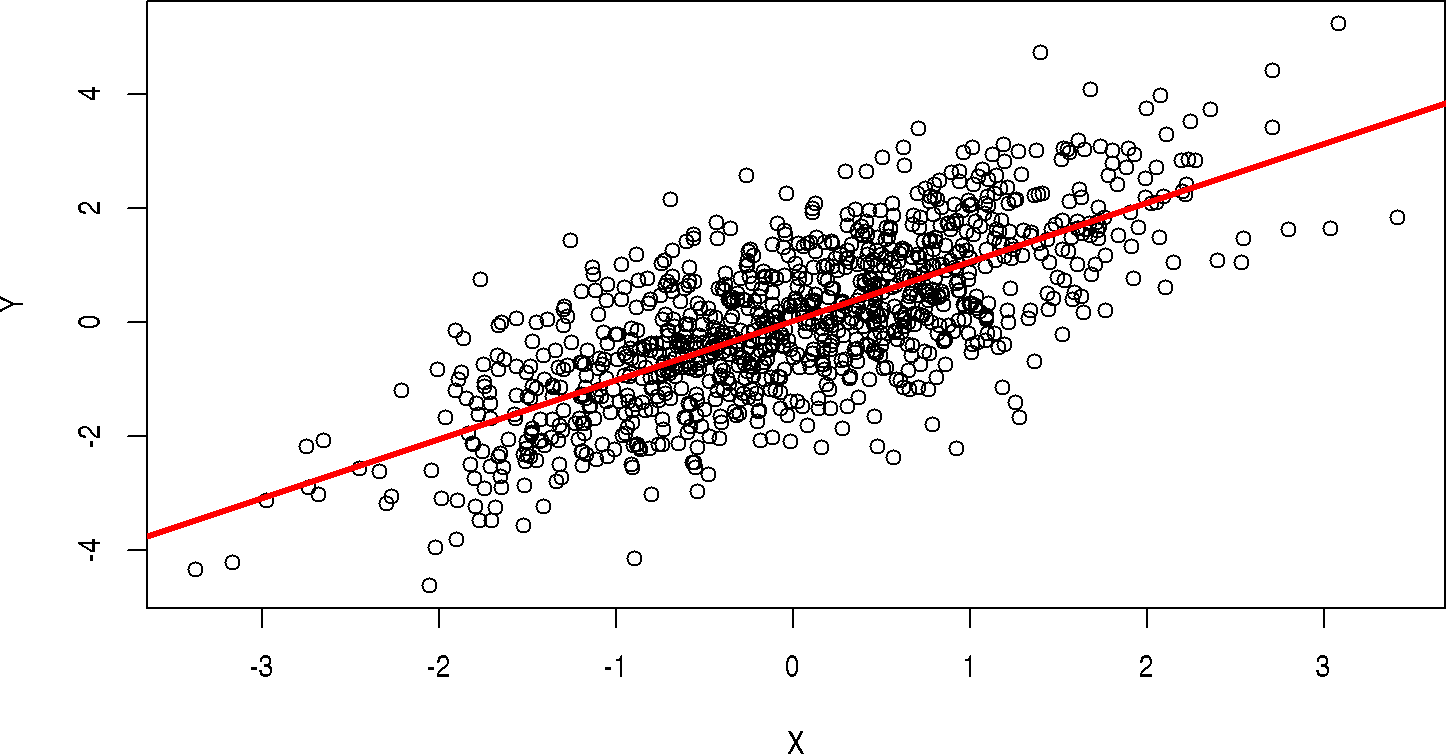# 1RStudio: A Quick Tour

Panes

Options

Help

Environment, History, and Files

# 2R: First Impressions

Type values and mathematical formulas into R’s command prompt

1 + 1
##  2

Assign values to symbols (variables)

x = 1
x + x
##  2

Invoke functions such as c(), which takes any number of values and returns a single vector

x = c(1, 2, 3)
x
##  1 2 3

R functions, such as sqrt(), often operate efficiently on vectors

y = sqrt(x)
y
##  1.000000 1.414214 1.732051

There are often several ways to accomplish a task in R

x = c(1, 2, 3)
x
##  1 2 3
x <- c(4, 5, 6)
x
##  4 5 6
x <- 7:9
x
##  7 8 9
10:12 -> x
x
##  10 11 12

Sometimes R does ‘surprising’ things that can be fun to figure out

x <- c(1, 2, 3) -> y
x
##  1 2 3
y
##  1 2 3

## 2.1R Data types: vector and list

‘Atomic’ vectors

• Types include integer, numeric (float-point; real), complex, logical, character, raw (bytes)

people <- c("Lori", "Nitesh", "Valerie", "Herve")
people
##  "Lori"    "Nitesh"  "Valerie" "Herve"
• Atomic vectors can be named

population <- c(Buffalo=259000, Rochester=210000, New York=8400000)
population
##   Buffalo Rochester  New York
##    259000    210000   8400000
log10(population)
##   Buffalo Rochester  New York
##  5.413300  5.322219  6.924279
• Statistical concepts like NA (“not available”)

truthiness <- c(TRUE, FALSE, NA)
truthiness
##   TRUE FALSE    NA
• Logical concepts like ‘and’ (&), ‘or’ (|), and ‘not’ (!)

!truthiness
##  FALSE  TRUE    NA
truthiness | !truthiness
##  TRUE TRUE   NA
truthiness & !truthiness
##  FALSE FALSE    NA
• Numerical concepts like infinity (Inf) or not-a-number (NaN, e.g., 0 / 0)

undefined_numeric_values <- c(NA, 0/0, NaN, Inf, -Inf)
undefined_numeric_values
##    NA  NaN  NaN  Inf -Inf
sqrt(undefined_numeric_values)
## Warning in sqrt(undefined_numeric_values): NaNs produced
##   NA NaN NaN Inf NaN
• Common string manipulations

toupper(people)
##  "LORI"    "NITESH"  "VALERIE" "HERVE"
substr(people, 1, 3)
##  "Lor" "Nit" "Val" "Her"
• R is a green consumer – recycling short vectors to align with long vectors

x <- 1:3
x * 2            # '2' (vector of length 1) recycled to c(2, 2, 2)
##  2 4 6
truthiness | NA
##  TRUE   NA   NA
truthiness & NA
##     NA FALSE    NA
• It’s very common to nest operations, which can be simultaneously compact, confusing, and expressive ([: subset; <: less than)

substr(tolower(people), 1, 3)
##  "lor" "nit" "val" "her"
population[population < 1000000]
##   Buffalo Rochester
##    259000    210000

Lists

• The list type can contain other vectors, including other lists

frenemies = list(
friends=c("Larry", "Richard", "Vivian"),
enemies=c("Dick", "Mike")
)
frenemies
## $friends ##  "Larry" "Richard" "Vivian" ## ##$enemies
##  "Dick" "Mike"
• [ subsets one list to create another list, [[ extracts a list element

frenemies
## $friends ##  "Larry" "Richard" "Vivian" frenemies[c("enemies", "friends")] ##$enemies
##  "Dick" "Mike"
##
## $friends ##  "Larry" "Richard" "Vivian" frenemies[["enemies"]] ##  "Dick" "Mike" Factors • Character-like vectors, but with values restricted to specific levels sex = factor(c("Male", "Male", "Female"), levels=c("Female", "Male", "Hermaphrodite")) sex ##  Male Male Female ## Levels: Female Male Hermaphrodite sex == "Female" ##  FALSE FALSE TRUE table(sex) ## sex ## Female Male Hermaphrodite ## 1 2 0 sex[sex == "Female"] ##  Female ## Levels: Female Male Hermaphrodite ## 2.2 Classes: data.frame and beyond Variables are often related to one another in a highly structured way, e.g., two ‘columns’ of data in a spreadsheet x = rnorm(1000) # 1000 random normal deviates y = x + rnorm(1000) # another 1000 deviates, as a function of x plot(y ~ x) # relationship between x and yConvenient to manipulate them together • data.frame(): like columns in a spreadsheet df = data.frame(X=x, Y=y) head(df) # first 6 rows ## X Y ## 1 0.4319017 -0.9987349 ## 2 0.8354825 0.5375856 ## 3 -1.6474644 -2.9015924 ## 4 -0.6308896 -1.1659811 ## 5 1.5897967 0.5246669 ## 6 2.7946085 1.6279334 plot(Y ~ X, df) # same as above• See all data with View(df). Summarize data with summary(df) summary(df) ## X Y ## Min. :-3.37638 Min. :-4.61834 ## 1st Qu.:-0.69195 1st Qu.:-0.87559 ## Median : 0.03476 Median : 0.03680 ## Mean : 0.02679 Mean : 0.05026 ## 3rd Qu.: 0.74620 3rd Qu.: 1.04719 ## Max. : 3.41133 Max. : 5.23651 • Easy to manipulate data in a coordinated way, e.g., access column X with $ and subset for just those values greater than 0

positiveX = df[df\$X > 0,]
head(positiveX)
##           X           Y
## 1 0.4319017 -0.99873488
## 2 0.8354825  0.53758557
## 5 1.5897967  0.52466689
## 6 2.7946085  1.62793340
## 8 0.6262119  0.05052979
## 9 1.0583540  1.68579650
plot(Y ~ X, positiveX)• R is introspective – ask it about itself

class(df)
##  "data.frame"
dim(df)
##  1000    2
colnames(df)
##  "X" "Y"
• matrix() a related class, where all elements have the same type (a data.frame() requires elements within a column to be the same type, but elements between columns can be different types).

A scatterplot makes one want to fit a linear model (do a regression analysis)

• Use a formula to describe the relationship between variables
• Variables found in the second argument

fit <- lm(Y ~ X, df)
• Visualize the points, and add the regression line

plot(Y ~ X, df)
abline(fit, col="red", lwd=3)• Summarize the fit as an ANOVA table

anova(fit)
## Analysis of Variance Table
##
## Response: Y
##            Df  Sum Sq Mean Sq F value    Pr(>F)
## X           1 1131.95    1132  1132.6 < 2.2e-16 ***
## Residuals 998  997.46       1
## ---
## Signif. codes:  0 '***' 0.001 '**' 0.01 '*' 0.05 '.' 0.1 ' ' 1
• N.B. – ‘Type I’ sums-of-squares, so order of independent variables matters; use drop1() for ‘Type III’. See DataCamp Quick-R

• Introspection – what class is fit? What methods can I apply to an object of that class?

class(fit)
##  "lm"
methods(class=class(fit))
##   add1           alias          anova          case.names
##   coerce         confint        cooks.distance deviance
##   dfbeta         dfbetas        drop1          dummy.coef
##  effects        extractAIC     family         formula
##  hatvalues      influence      initialize     kappa
##  labels         logLik         model.frame    model.matrix
##  nobs           plot           predict        print
##  proj           qr             residuals      rstandard
##  rstudent       show           simulate       slotsFromS3
##  summary        variable.names vcov
## see '?methods' for accessing help and source code

## 2.3 Help!

Help available in Rstudio or interactively

• Check out the help page for rnorm()

?rnorm
• ‘Usage’ section describes how the function can be used

rnorm(n, mean = 0, sd = 1)
• Arguments, some with default values. Arguments matched first by name, then position

• ‘Arguments’ section describes what the arguments are supposed to be

• ‘Value’ section describes return value

• ‘Examples’ section illustrates use

• Often include citations to relevant technical documentation, reference to related functions, obscure details

• Can be intimidating, but in the end actually very useful